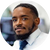# How do I select a random item from a JavaScript array?

Hello CodeNewbie! I'm teaching myself JavaScript right now and am having a hard time with selecting a random item from a JavaScript array. Can anyone help illuminate the issue for me?

Cheers!Byron Jenkins

Hello Harry.

So array indices go from 0 to array.length - 1. When selecting a random item, we want to get a random integer (whole number) within that range.

Knowing this, we can define a function `getRandomElement` that takes in an array and returns a random element from the given array. I defined it as a function assuming you would use this more than once. It's good practice to do this to avoid repeating code (DRY: Don't Repeat Yourself).

``````function getRandomElement(arr) {
// gets number from to 0 to arr.length - 1 inclusive
let index = Math.floor(Math.random() * arr.length);
return arr[index];
}

// Example usage
let fruits = ["apple", "orange", "banana", "mango"];
let randomFruit = getRandomElement(fruits);
console.log(randomFruit);
``````

Resources:
Math.floor
Math.random